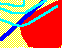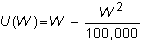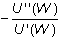7.20 CHAPTER 7: EXERCISES

In the following exercises an (*) denotes greater difficulty.

1a) What is the maximum amount you are willing to pay to participate in the St Petersburg game?

1b) Suppose you had the utility function defined over wealth that equals 1+ln(W) where ln is a natural (to the base e) logarithm and W is wealth.

What would be the maximum amount you would pay to play this game?

If you cannot calculate the value directly, compute it on a spreadsheet for 100 throws.

2. Suppose an investor has the following utility function defined over wealth.and must choose between the following two risky mutually exclusive alternatives (i.e., only one can be selected).

Alternative A

 Payoff Probability -\$200 1/3 +\$300 1/3 +\$500 1/3

Alternative B

 Payoff Probability -\$50 5/10 +\$250 4/10 +\$1000 1/10

a) Would you choose one alternative over the other? Explain your answer.

Suppose that alternative B was changed to the following:

Alternative B

 Payoff Probability -\$50 5/10 +\$300 4/10 +\$900 1/10

c) To what would you have to change the constant in the utility function from 100,000 to reverse your answer in b)?

3a) If you became wealthier do you think that you would become more or less risk averse?

3b) The Arrow Pratt measure of risk aversion for utility function U(W) is:i) For the utility function, U(W) = W - kW2 show that this measure is increasing in W which implies that as you get wealthier you get more risk averse.

ii) For the utility function U(W) = A - e-kx, show that risk aversion is independent of wealth.

iii) For the utility function, U(W) = ln(W), show that risk aversion decreases with wealth.

4. Use CAPM Tutor's Market Equilibrium subject for this question.

Click on the tangency point (M, shown in default colors as the green point) between the CML and the minimum variance frontier, to see the indifference curve of the "average investors" indifference curve. Note that this investor puts all their wealth into the market portfolio.

Click on the point on the CML where an investor holds 50% of their wealth in the market portfolio and 50% of their wealth in the risk-free security. Repeat this for an investor that holds 150% in the market portfolio and borrows 50% at the risk-free rate of interest.

a) Describe the relationship between the shape of the indifference curve and the investors attitude toward risk and return.

b) Explain how this attitude translates into portfolio choice.

5. Use CAPM Tutor's Market Equilibrium subject for this question.

a) If you increase the average risk aversion, what happens to the slope of the CML?

b) What does this imply for the market's tradeoff between risk and return?

c) When you increase the average risk aversion in the market, are investors better off or worse off?

d) What happens to the riskiness of each security, measured by the beta?

6. Use CAPM Tutor's Market Equilibrium subject for this question.

If you increase the risk free interest rate from 12% to 15%

a) What happens to the risk and the return on the market portfolio?

b) Explain what happens to the market price of risk?

c) What happens to the riskiness of each security, measured by the beta?

7. Explain what is meant by the statement "the CAPM is essentially untestable."

previous topic

next topic